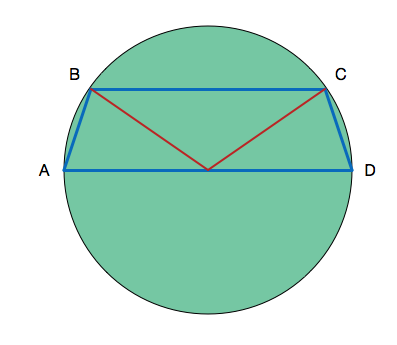# I've seen it before - 3

Geometry Level 3If $ABCD$ is an isosceles trapezium inscribed in a semi-circle with diameter $AD$ and $AB=CD=2\text{ cm}$ and the radius of the semi-circle is $4 \text{ cm}$, then the length of $BC$ (in cm) is:

×